# Understanding inductive kickback in boost converter

• srinaath
Now consider normal boost converter with dc source voltage, inductor, switch, diode and load resistor...when switch is ON then inductor polarity will be shown in fig...when switch is OFF inductor polarity should be changed (due to kickback) ..this causes the diode to conduct..When SW1 is turned off, the inductor voltage will be negative. This causes current to flow out of the inductor and into the load.f

#### srinaath

please correct me if i am worng with the understading of inductive kickback,"voltage polarity across inductor will be such that it opposes the source voltage"...
inductive kickback occurs when there is sudden change in current through the inductor... in that case inductor voltage will go very high...
now consider normal boost converter with dc source voltage, inductor, switch, diode and load resistor...when switch is ON then inductor polarity will be shown in fig...when switch is OFF inductor polarity should be changed (due to kickback) ..this causes the diode to conduct..
1)do inductive kickback occurs for short duration of time?
2)in analyzing boost converter, polarity of inductor is kept constant for both modes of operations...why is that so?
3)the purpose of diode is to overcome inductive kickback?? (am i right??)

inductive kickback occurs when there is sudden change in current through the inductor.
Inductive voltage flyback or kickback occurs when current has no other way to flow. V = L * di / dt.

You need to attach a circuit diagram before asking specific questions about circuit behaviour.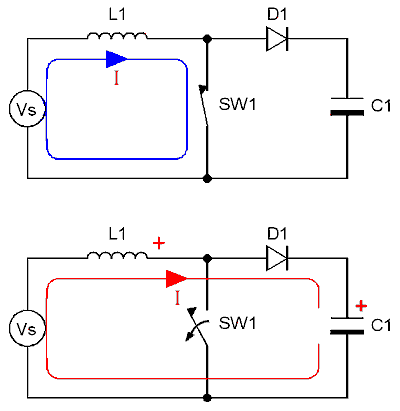hi baluncore, this is my circuit...

Inductive kickback is usually restricted to the destructive voltage spike that occurs when turning off an inductive load.

The circuit has two states, decided by the position of SW1.
While SW1 conducts, voltage across L1 is positive, so current flow in L1 rises. Vs = L * di / dt
While D1 conducts, voltage across L1 is negative so current flow in L1 falls. (Vs – Vc1) = L * di / dt

The current always flows the same direction through L1 but the polarity of L1 voltage reverses.

When current flows uphill through L1 into C1, I believe you are calling that inductive kickback.
There is a voltage step from ground to Vc1 when SW1 turns off.
There is no high voltage spike because it is clamped by D1 into C1.

D1 is an automatic switch that blocks current flow from C1 through SW1 to ground.
D1 automatically conducts when SW1 does not. It allows L1 current to charge C1.

Inductive kickback is usually restricted to the destructive voltage spike that occurs when turning off an inductive load.

The circuit has two states, decided by the position of SW1.
While SW1 conducts, voltage across L1 is positive, so current flow in L1 rises. Vs = L * di / dt
While D1 conducts, voltage across L1 is negative so current flow in L1 falls. (Vs – Vc1) = L * di / dt

The current always flows the same direction through L1 but the polarity of L1 voltage reverses.

When current flows uphill through L1 into C1, I believe you are calling that inductive kickback.
There is a voltage step from ground to Vc1 when SW1 turns off.
There is no high voltage spike because it is clamped by D1 into C1.

D1 is an automatic switch that blocks current flow from C1 through SW1 to ground.
D1 automatically conducts when SW1 does not. It allows L1 current to charge C1.

hmm...understood a bit...but still have doubt...say switch SW1 is closed, at that time inductor gets charged, but why not diode can conduct at that time?...please clarify me...

say switch SW1 is closed, at that time inductor gets charged, but why not diode can conduct at that time?
The diode can only conduct current in one direction. It is a one way valve.
While SW1 conducts the diode has reversed voltage so it does not conduct.
https://en.wikipedia.org/wiki/P–n_diode

The diode can only conduct current in one direction. It is a one way valve.
While SW1 conducts the diode has reversed voltage so it does not conduct.
https://en.wikipedia.org/wiki/P–n_diode
thanks...baluncore...why is that during analysis the polarity of inductor is kept same, I mean when SW1 is closed inductor has + - polarity but when SW1 is opened, inductor has -+ polarity (thats y diode comes into picture)...but during both switching modes inductor polarity is kelp +- in many books...can you please clarify this also..thanks

...but during both switching modes inductor polarity is kelp +- in many books...
They might be more common buck converters that reduce the voltage. Post an example and I might be able to comment.
Your circuit is for a boost converter that always delivers to a greater voltage.

but during both switching modes inductor polarity is kelp +- in many books
Do you mean something like this ?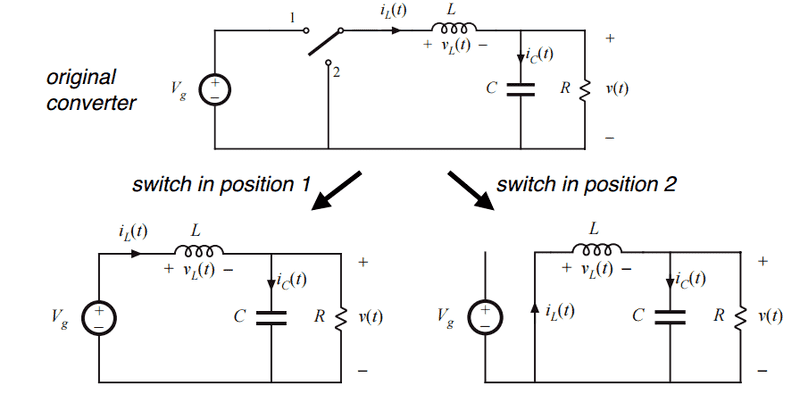The author did a good job in confusing the beginners.This is a very bad habit to show "pre-analysis polarities". The polarity of inductor voltage is what he (the author) decide to choose before solving the circuit. So this diagram did not show "actual polarities".

And remember one thing:
The voltage across an ideal inductor is VL = L* ΔI/Δt
This equation indicates that inductance voltage depends not on current which actually flows through the inductance, but on its rate of change. This means that to produce the voltage across an inductance, the applied current must change. If the current is kept constant, no voltage will be induced, no matter how large the current. Conversely, if it is found that the voltage across an inductance is zero this means that the current must be constant but not necessary zero.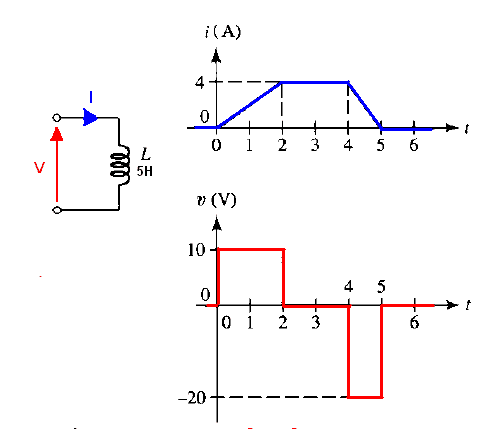In summary:
When the current is increasing di/dt > 0, so V must be positive because L times a positive number yields a positive voltage.
When the current is decreasing di/dt < 0, so V must be negative because L times a negative number yields a negative voltage.
When we have no change in current over time then we can't have any voltage V = L*di/dt = L * 0 = 0.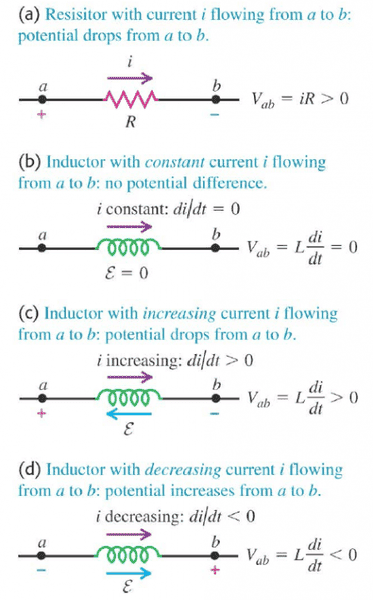http://booksite.elsevier.com/samplechapters/9780750679701/9780750679701.PDF (from page 22 Understanding the Inductor)

Sample
Why is there any voltage even present across the inductor? We always accept a voltage across a resistor without argument because we know Ohm’s law (V = I × R) all too well. But an inductor has (almost) no resistance it is basically just a length of solid conducting copper wire (wound on a certain core). So how does it manage to “hold-off” any voltage across it?
In fact, we are comfortable about the fact that a capacitor can hold voltage across it. But for the inductor, we are not very clear!
A mysterious electric field somewhere inside the inductor! Where did that come from?
It turns out, that according to Lenz and/or Faraday, the current takes time to build up in an inductor only because of ‘induced voltage.’ This voltage, by definition, opposes any external effort to change the existing flux (or current) in an inductor. So if the current is fixed, yes, there is no voltage present across the inductor, it then behaves just as a piece of conducting wire. But the moment we try to change the current, we get an induced voltage across it. By definition, the voltage measured across an inductor at any moment (whether the switch is open or closed) is the ‘induced voltage.’
So let us now try to figure out exactly how the induced voltage behaves when the switch is closed. Looking at the inductor charging phase, the inductor current is initially zero. Thereafter, by closing the switch, we are attempting to cause a sudden change in the current. The induced voltage now steps into try to keep the current down to its initial value(zero).
So we apply ‘Kirchhoff’s voltage law’ to the closed loop in question. Therefore, at the moment the switch closes, the induced voltage must be exactly equal to the applied voltage, since the voltage drop across the series resistance R is initially zero (by Ohm’s law).
As time progresses, we can think intuitively in terms of the applied voltage “winning.” This causes the current to rise up progressively. But that also causes the voltage drop across R to increase, and so the induced voltage must fall by the same amount (to remain faithful to Kirchhoff’s voltage law).
That tells us exactly what the induced voltage (voltage across inductor) is during the entire switch-closed phase.
Why does the applied voltage “win”? For a moment, let’s suppose it didn’t. That would mean the applied voltage and the induced voltage have managed to completely counter-balance each other — and the current would then remain at zero. However, that cannot be, because zero rate of change in current implies no induced voltage either! In other words, the very existence of induced voltage depends on the fact that current changes, and it must change.
We also observe rather thankfully, that all the laws of nature bear each other out. There is no contradiction whichever way we look at the situation. For example, even though the current in the inductor is subsequently higher, its rate of change is less, and therefore, so is the induced voltage (on the basis of Faraday’s/Lenz’s law). And this “allows” for the additional drop appearing across the resistor, as per Kirchhoff’s voltage law!

On of the best resources is the TI PS Topologies Poster

Here you see the waveform for the various elements -

I also like to point out that inductor is essentially the opposite of a Cap. Vind = L dI/dT vs Icap = C dV/dT. When you charge a cap current flows "in" one direction and then with the same polarity of voltage the current can reverse and flow out. The inductor - the current can be the same polarity but the voltage changes polarity,

Next -- what is happening?, we are storing energy(power input) in the inductor while the switch is closed, and the inductor is supplying energy (power output) when it is open, To change the Direction of power flow ether voltage or current must change polarity. In a Cap the current changes - in an Inductor it is the Voltage.

It is easy to get in the habit of drawing ladder diagrams for analysis of circuits. But ladders are view best suited to a mathematical analysis. The TI poster referenced above in post #10 uses ladder diagrams.

When trying to show or understand quickly how a circuit works, I prefer to keep high voltages at the top of the diagram with low at the bottom. The source or input is on the left with the load or output on the right. Attached is a diagram showing buck and boost converters as an example.

Notice that the two alternate switch paths are placed parallel in line. The “inherent” switch diodes are shown with an orientation as reverse biassed to make their use clear. You will also better notice the mirror symmetry of topology in the step down and step up circuits.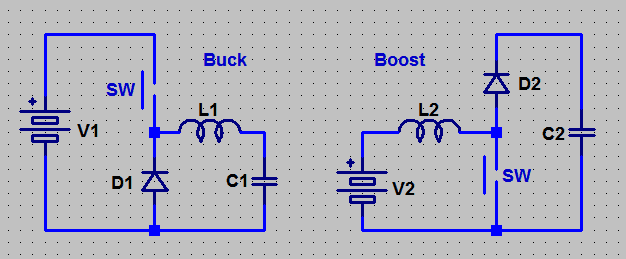Do you mean something like this ?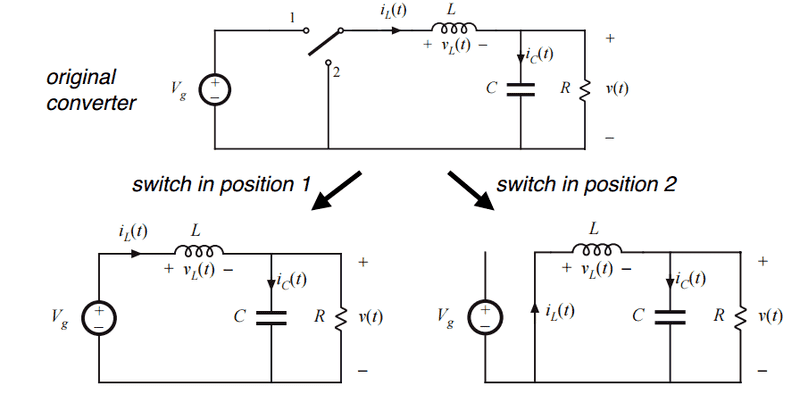•